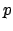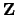## A Method for Factoring that Always Works

There are numbers fieldssuch thatis not of the formfor any. Even worse, Dedekind found a fieldsuch thatfor all, so there is no choice ofsuch that Theorem 8.1.3 can be used to factorfor(see Example 8.1.6 below).

Most algebraic number theory books do not describe an algorithm for decomposing primes in the general case. Fortunately, Cohen's book [Coh93, §6.2]) describes how to solve the general problem. The solutions are somewhat surprising, since the algorithms are much more sophisticated than the one suggested by Theorem 8.1.3. However, these complicated algorithms all run very quickly in practice, even without assuming the maximal order is already known.

For simplicity we consider the following slightly easier problem whose solution contains the key ideas: Letbe any order inand letbe a prime of. Find the prime ideals ofthat contain.

To go from this special case to the general case, given a primethat we wish to factor in, we find a-maximal order, i.e., an ordersuch thatis coprime to. A-maximal order can be found very quickly in practice using the round 2'' or round 4'' algorithms. (Remark: Later we will see that to compute, we take the sum of-maximal orders, one for everysuch thatdivides. The time-consuming part of this computation ofis finding the primessuch that, not finding the-maximal orders. Thus a fast algorithm for factoring integers would not only break many cryptosystems, but would massively speed up computation of the ring of integers of a number field.)

Algorithm 8.1.4   Supposeis an order in the ringof integers of a number field. For any prime, the following (sketch of an) algorithm computes the set of maximal ideals ofthat contain.

Sketch of algorithm. Letbe a number field given by an algebraic integeras a root of its minimal monic polynomialof degree. We assume that an orderhas been given by a basisand thatthat contains. Each of the following steps can be carried out efficiently using little more than linear algebra over. The details are in [Coh93, §6.2.5].

1. [Check if easy] If(so), then by a slight modification of Theorem 8.1.3, we easily factor.
2. [Compute radical] Letbe the of, which is the ideal of elementssuch thatfor some positive integer. Using linear algebra over the finite field, we can quickly compute a basis for. (We never compute.)
3. [Compute quotient by radical] Compute anbasis forThe second equality comes from the fact that, which is clear by definition. Note thatis obtained by simply reducing the basismodulo.
4. [Decompose quotient] The ringis a finite Artin ring with no nilpotents, so it decomposes as a productof fields. We can quickly find such a decomposition explicitly, as described in [Coh93, §6.2.5].
5. [Compute the maximal ideals over] Each maximal ideallying overis the kernel of.

The algorithm finds all primes ofthat contain the radicalof. Every such prime clearly contains, so to see that the algorithm is correct, we must prove that the primesofthat containalso contain. Ifis a prime ofthat contains, then. Ifthenfor some, sowhich implies thatby primality of. Thuscontains, as required.

William Stein 2004-05-06